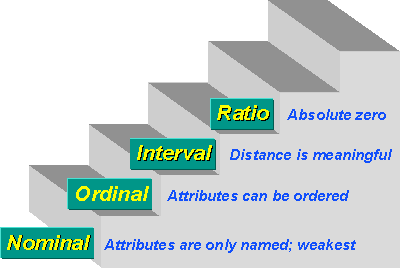# Measurement scales: Nominal, Ordinal, Interval, and Ratio

Measurement scale, in statistical analysis, the type of information provided by numbers. Each of the four scales (i.e., nominal, ordinal, interval, and ratio) provides a different type of information. Measurement refers to the assignment of numbers in a meaningful way, and understanding measurement scales is important to interpreting the numbers assigned to people, objects, and events.### Nominal Scales

In nominal scales, numbers, such as driver’s license numbers and product serial numbers, are used to name or identify people, objects, or events. Gender is an example of a nominal measurement in which a number (e.g., 1) is used to label one gender, such as males, and a different number (e.g., 2) is used for the other gender, females. Numbers do not mean that one gender is better or worse than the other; they simply are used to classify persons. In fact, any other numbers could be used, because they do not represent an amount or a quality. It is impossible to use word names with certain statistical techniques, but numerals can be used in coding systems. For example, fire departments may wish to examine the relationship between gender (where male = 1, female = 2) and performance on physical-ability tests (with numerical scores indicating ability).

Ordinal Scales

In ordinal scales, numbers represent rank order and indicate the order of quality or quantity, but they do not provide an amount of quantity or degree of quality. Usually, the number 1 means that the person (or object or event) is better than the person labeled 2; person 2 is better than person 3, and so forth—for example, to rank order persons in terms of potential for promotion, with the person assigned the 1 rating having more potential than the person assigned a rating of 2. Such ordinal scaling does not, however, indicate how much more potential the leader has over the person assigned a rating of 2, and there may be very little difference between 1 and 2 here. When ordinal measurement is used (rather than interval measurement), certain statistical techniques are applicable (e.g., Spearman’s rank correlation).

Interval Scale

In interval scales, numbers form a continuum and provide information about the amount of difference, but the scale lacks a true zero. The differences between adjacent numbers are equal or known. If zero is used, it simply serves as a reference point on the scale but does not indicate the complete absence of the characteristic being measured. The Fahrenheit and Celsius temperature scales are examples of interval measurement. In those scales, 0 °F and 0 °C do not indicate an absence of temperature.

Ratio Scales

Ratio scales have all of the characteristics of interval scales as well as a true zero, which refers to complete absence of the characteristic being measured. Physical characteristics of persons and objects can be measured with ratio scales, and, thus, height and weight are examples of ratio measurement. A score of 0 means there is complete absence of height or weight. A person who is 1.2 metres (4 feet) tall is two-thirds as tall as a 1.8-metre- (6-foot-) tall person. Similarly, a person weighing 45.4 kg (100 pounds) is two-thirds as heavy as a person who weighs 68 kg (150 pounds).

## 2 thoughts on “Measurement scales: Nominal, Ordinal, Interval, and Ratio”

error: Content is protected !!